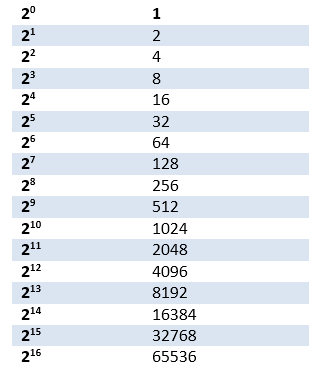# The single thing about binary that every developer should know about – No excuse

There are many things about bits and binary system that each developer should know. However I’m not talking in this article about the trivial things (such as how many bits are in a byte, or how many bytes are in a kilobyte) … nor I will talk about advanced stuff such as bit manipulation or advanced bitwise algorithms such as error detection and correction codes.

Instead I will focus on a very elementary thing that each and every software developer should know about. This boils down to two questions:

• Q1: What is the maximum natural number that you can represent on n bits?

… and the inverse …

• Q2: Given a certain number x, what is the minim number of bits that are needed to represent all the natural numbers up to that number?

Although elementary, these questions are the base of understanding binary and data types in various programming languages.

At this point I will address you, the reader. If you are the impatient type or if you believe you know already the answer to these questions, just jump ahead to the last section of this article, the one with the design problem.

## Q1: What is the maximum natural number that you can represent on n bits?

Generically speaking with n bits available you can represent 2^n possible values. These values have the meaning that the designer of the data structure intended to give them.

In this case, we find out by reading the question carefully, that in the bit based data structure we want to represent natural numbers such as 0, 1, 2, …, x. We can safely assume that all the bits are used only to represent these numbers like this: first value is used to represent number 0, the second value to represent number 1, etc.

Therefore, to answer the question, the maximum natural number that can be represented on n bits is 2^n – 1.

As you can see in the formula, obtaining the maximum number can be calculated simply by subtracting 1 from 2^n. Knowing power of 2 for common n values comes handy in many other situations, therefore any developers should be familiar with the most commonly encountered powers of 2:Note: The answer to Q1 is totally different if the designer wants to store also negative and / or decimal numbers by giving different meaning to various bits in the data structure.

## Q2: Given a certain natural number x, what is the minim number of bits n that are needed to represent x?

From the beginning we can see that this question is the inverse of the previous one.

One may therefore try to raise number 2 to successive powers until the result is equal or bigger than x. At that moment n was found.

However, you don’t have to do all these calculations if you remember from school about logarithms. Quick refresher: if 2^n = x then n = log2(x)

With this new tool, figuring out n when you know x, is a matter of calculating log2(x).

Depending on the situation, you can quickly calculate log2 with a pocket calculator, with Google or even inside your algorithm. For instance you can do this in JavaScript:

``````var n = Math.ceil( Math.log2( x + 1 ) );
``````

Note the +1 adjustment to compensate for zero (since x is a natural number).

## Design problem

Before closing the article, let me give you a quick design problem:

You want to design a timestamp like structure to record the time, with millisecond accuracy, for the next 100 years. How many bits do you need to do this?

First we need to figure out approximately how many milliseconds are in 100 years. Since we try to simplify the calculations, we will be generous and approximate all years to 366 days.

Therefore MS = 100 * 366 * 24 * 3600 * 1000 = 3,162,240,000,000 milliseconds in 100 years.

By doing log2 on the above number and rounding to the appropriate integer value, we find out the answer: 42.

Therefore we need 42 bits to represent any date/time stamp for the next 100 years with an accuracy of millisecond.

Therefore we can use for this job a 64 bit data type (e.g. register, variable, etc.). For instance a C# ulong will be the perfect candidate. Since we don’t even need the whole 64 bits, we may even decide to use the remaining 22 bits in the data structure for representing other kind of information besides the timestamp.

But what if all the 64 bits in the ulong variable will be used to represent the timestamp? What is the maximum number of years that a 64 bit variable can represent with millisecond accuracy?

The calculation is as easy as before, only done in reverse:

2 ^ 64 / 1000 / 3600 / 24 / 366 which is more than 583 million years! Wow!

Don’t forget to check my other article for more wow facts about the 64 bits structures.